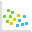#Scatter plot

Plots the occurences of two number variables in a scatter plot chart. A useful visualization for identifying outliers in numeric data relationships.

Analyzer

## Configured properties

• ### Group column

InputColumn<Object> Optional
• ### Variable1

The field with the first variable. Will be plotted on the horizontal X-axis.

InputColumn<Number> Required
• ### Variable2

The field with the second variable. Will be plotted on the vertical Y-axis.

InputColumn<Number> Required